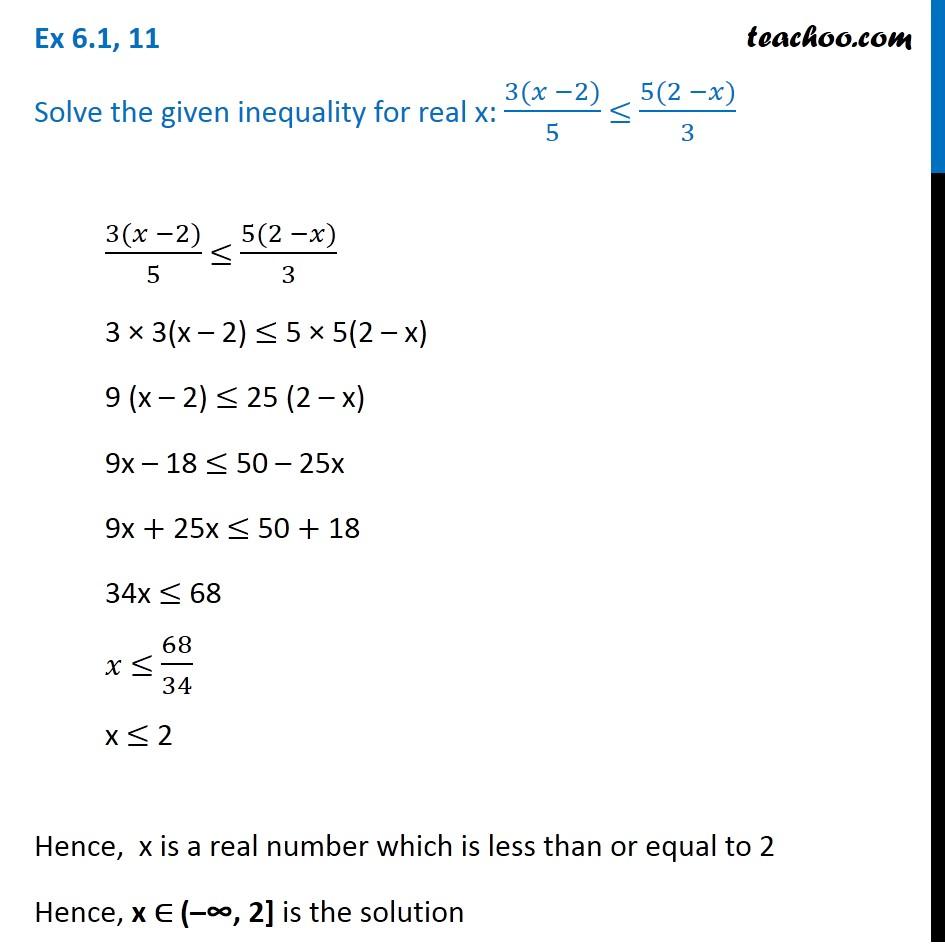Ex 5.1

Chapter 5 Class 11 Linear Inequalities
Serial order wiseLearn in your speed, with individual attention - Teachoo Maths 1-on-1 Class

### Transcript

Ex 5.1, 11 Solve the given inequality for real x: (3(𝑥 −2))/5 ≤ (5(2 −𝑥))/3 (3(𝑥 −2))/5 ≤ (5(2 −𝑥))/3 3 × 3(x – 2) ≤ 5 × 5(2 – x) 9 (x – 2) ≤ 25 (2 – x) 9x – 18 ≤ 50 – 25x 9x + 25x ≤ 50 + 18 34x ≤ 68 𝑥 ≤ 68/34 x ≤ 2 Hence, x is a real number which is less than or equal to 2 Hence, x ∈ (–∞, 2] is the solution Ex 5.1, 11 Solve the given inequality for real x: 3( 2) 5 5(2 ) 3 3( 2) 5 5(2 ) 3 3 3(x 2) 5 5(2 x) 9 (x 2) 25 (2 x) 9x 18 50 25x 9x + 25x 50 + 18 34x 68 68 34 x 2 Hence, x is a real number which is less than or equal to 2 Hence, x ( 2] is the solution